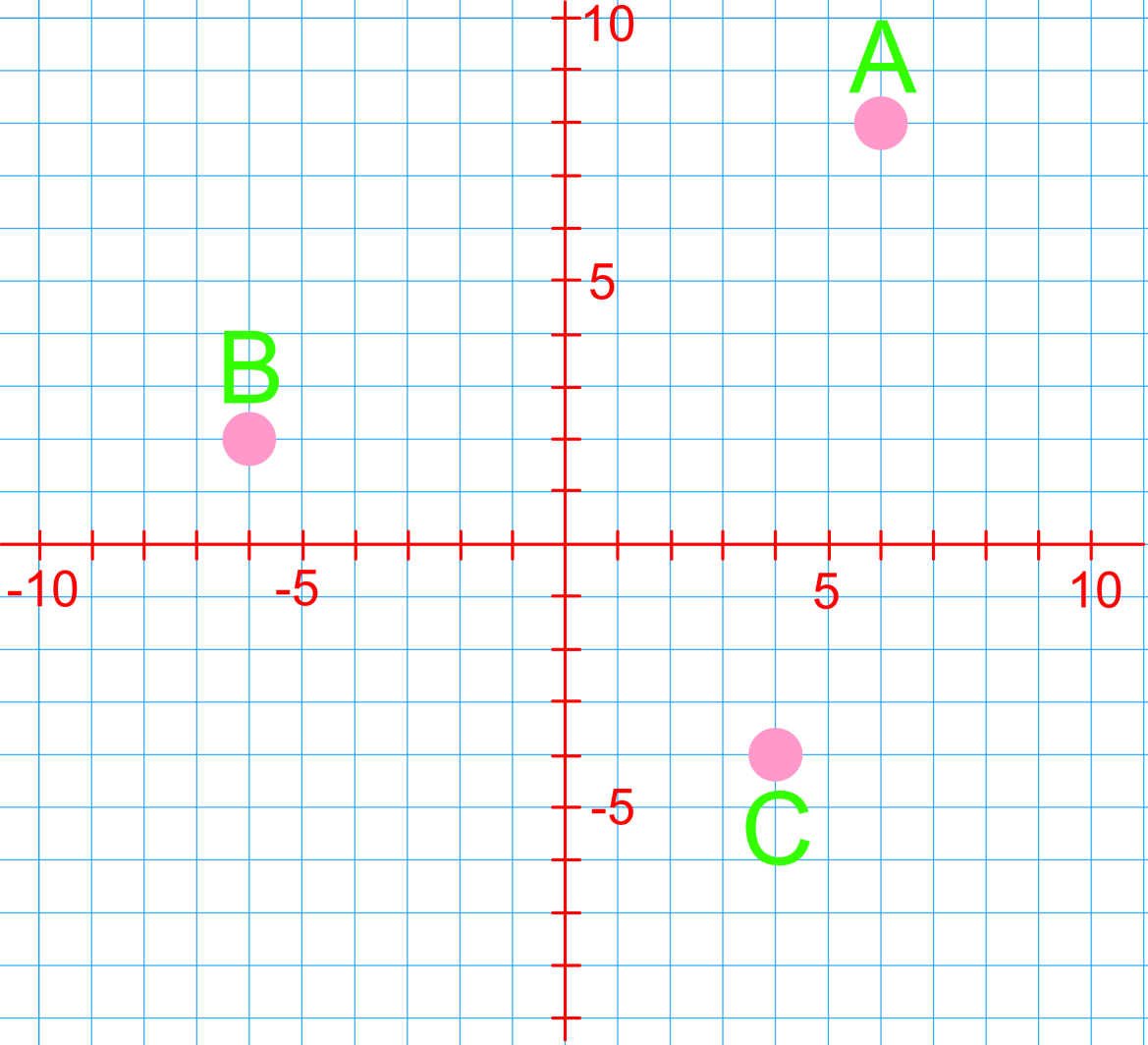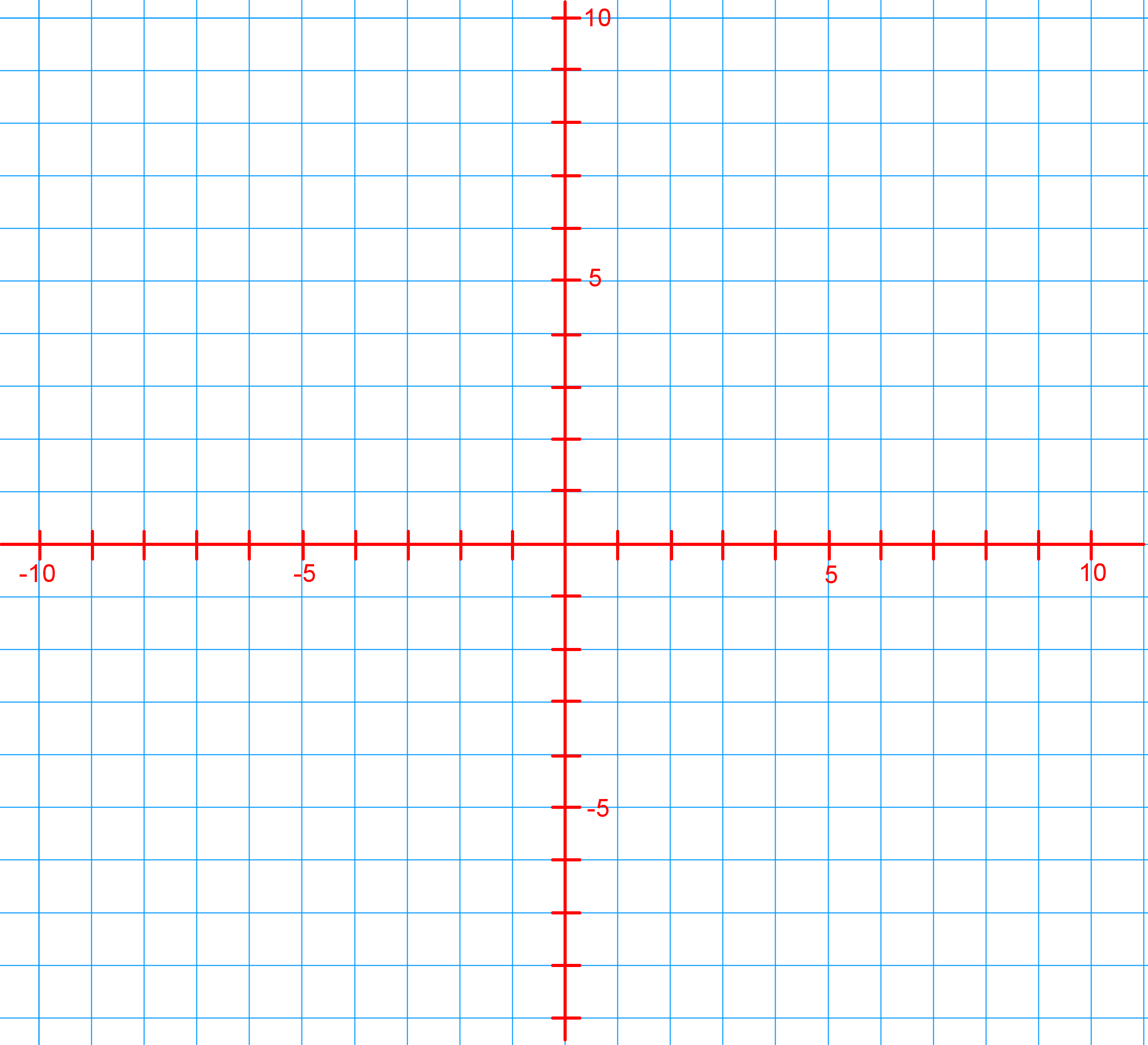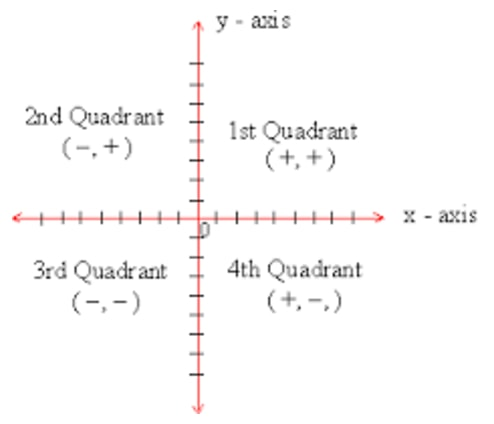# Cartesian plane#### Everything You Need in One Place

Homework problems? Exam preparation? Trying to grasp a concept or just brushing up the basics? Our extensive help & practice library have got you covered.#### Learn and Practice With Ease

Our proven video lessons ease you through problems quickly, and you get tonnes of friendly practice on questions that trip students up on tests and finals.#### Instant and Unlimited Help

Our personalized learning platform enables you to instantly find the exact walkthrough to your specific type of question. Activate unlimited help now!

0/1
##### Intros
###### Lessons
1. Introduction to x$-$y plane
0/3
##### Examples
###### Lessons
1. What are the coordinates of each point shown on the coordinate grid?1. Predict in which quadrant each of the following points will lie. Then, plot the points on a coordinate grid: A (4, -1), B (-7, 3), C (-2, -5), D (0, 2), E (-5, 0)1. Maggie walks to the pool every evening. Her house lies at H (-9, 0) and the pool lies at P (9, 0). Join the pair of coordinates with a straight line segment. What is the total distance from her house to the pool? Each grid line/square represents 1km.0%
##### Practice
###### Topic Notes
Captains of a ship must plot their ship's location and destination points on a grid. Similar to a captain, in this section we will learn how to label and plot coordinates on a given grid. In a coordinate grid, the horizontal number line is called the x-axis and the vertical number line is called the y-axis. These x and y axes meet at a point called the origin with coordinates (0, 0). The x and y axes are similar to the horizontal and vertical number lines we used in previous sections when learning how to add and subtract integers. When plotting coordinates, we always start at the origin. First, we count x units left or right from the origin. Next, we count y units up or down.

## Cartesian Coordinates System

The Cartesian plane was created by René Descartes to help people identify where something was located on a map or a graph. It uses a relationship between two variables. What are the elements to a Cartesian Coordinates System? Let's find out!

## X and Y Axis

The main way that the Cartesian Coordinates System allows you to locate something is through its x and y axis. The x axis is what you call the left-right direction of the plane. A way to help you remember this is that "x" is a cross. Therefore, x goes "across" on the Cartesian plane. The y axis is what you call the up-down direction. A Cartesian plane will always have both the x and y axis.

When you write down a pair of coordinates to help other people locate something on a plane, you'll have to write it in a specific way. Keep in mind that it always comes in a pair since there's the x and y axis that you'll have to consider. This is also called an ordered pair.

There is a specific way you're supposed to write them and it's that you write the horizontal distance before the vertical one. Therefore, an ordered pair looks like this: (x, y).

You may come across the terms "axis of ordinates" and "axis of abscissae". The ordinate simply refers to the vertical portion of an ordered pair, that is, the y axis. The abscissae refers to the horizontal part of a coordinate, this is, the x axis.

A Cartesian plane's x and y axis divides up the plane into four quadrants. Quadrant I is located where x and y is positive (the top right corner of the plane). Quadrant II is where x is negative but y is positive (the top left corner of the plane). Quadrant III is where both x and y are negative (the bottom left corner). Lastly, quadrant IV is where x is positive and y is negative (the bottom right corner).

You may be asked to identify which quadrant a set of coordinates lie in, or be told that an ordered pair is in a certain quadrant. Let's try out some practice problems to see how coordinates work on a Cartesian plane.

## Example problems

Question 1

What are the coordinates of each point shown on the coordinate grid?

Solution:

A=(ordinate, abscissa)= (x,y)= (6,8)

B=(ordinate, abscissa)= (x,y)= (-6,2)

C=(ordinate, abscissa)= (x,y)= (4,-4)

Question 2

Predict in which quadrant each of the following points will lie. Then, plot the points of a coordinate grid: A (4,-1), B (-7,3), C (-2,-5), D (0,2), E (-5,0)

Solution:The signs of x and y coordinates and where the points lie

Question 3

Maggie walks to the pool every evening. Her house lies at H(-9,0) and the pool lies at P (9,0)

Join the pair of coordinate with a straight line segment. What is the total distance from her house to the pool? Each grid line/square represents 1km.

Solution: